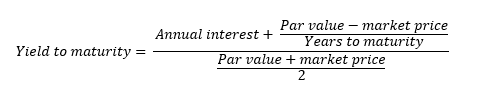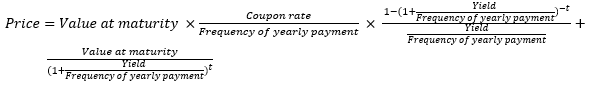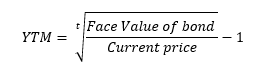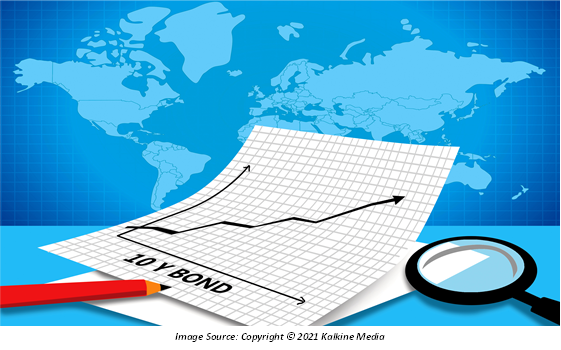# Yield to Maturity (YTM)

• Updated on

## What is yield?

Yield is the percentage of return from an investment over a specified time interval. It includes interest or dividend received during the investment period. There is a difference between yield and total return. Generally, yield refers to the income while the total return is defined as the capital gains, distribution, interest, and dividend received.

## What is Yield to Maturity (YTM)?

YTM determines the return an investor gains if the bond is held onto till it matures. YTM comprises of all interest payments including interest on interest received.

YTM can be considered the IRR (internal rate of return) on a bond and is expressed as an annual rate.

YTM assumes that the security is purchased at the current market price and will be held until maturity. Moreover, the interest received is reinvested at a constant rate.

## What is the formula for YTM?

While calculating YTM, maturity value, coupon payment and current price of bond are known to the investor.Calculating YTM with trial and error method involves the present value formula for calculating bond price –YTM formula on discount bond (Does not pay coupon rate) is –Here, t = years to maturity

## Summary

• YTM determines the return an investor gains if the bond is held onto its maturity. YTM comprises of all interest payment including interest on interest received.
• YTM is like the Internal Rate of Return (IRR) on a bond.
• YTM is also known as redemption yield or book yield.
• Trial and error method is best method for calculating YTM.

## What are the uses of YTM?

YTM is used by the investors to determine whether the bond they are buying is a good investment or not. For analysing a particular bond, the investor determines their required rate of return and compares it with the YTM.

Since YTM is expressed in the annual rate, it allows investors to conduct a comparison between different bonds as the time to maturity is ignored.

With YTM, comparison can also be conducted between different securities and bonds in terms of the return.

There is an inverse relationship between yield and market price of bonds, that is, a rise in the price of bond results in a fall in the yield of a bond and vice versa. Therefore, YTM allows an investor to understand the changing bond price due to changing market conditions.## What are the limitations of YTM?

YTM calculations ignore the cost of selling and buying a bond.

YTM calculation does not incorporate the taxes that an investor must pay while making investments in bonds.

The assumptions of YTM act as limitations. For example, YTM assumes that interest received is reinvested at a constant rate. However, there is a possibility that the investor is not able to re-invest at the interest received. Moreover, it might not be possible for an investor to hold onto a bond till its maturity.

## What are the variations or types of yield to maturity?

Variations can be observed in YTM due to the embeddedness of options.

Yield to call: It assumes that the issuer might purchase back the issued bond before it reaches maturity, resulting in a shorter period of cash flow. The calculation is altered by estimating the time when the bond can be repurchased.

Yield to put: Like YTC, it assumes that the holder of bond can sell the bond to the issuer at the price pre-determined while issuing the put bond.

Yield to worst: It has components of both YTC and YTP, that is, the issuer can buy back or holder can sell to the issuer any time it is financially feasible for either party.

## How is YTM different from coupon rate?

Coupon rate stands for the interest payment received by an investor till the maturity of bond. It a percentage of an annual rate of interest on the face value of bond.

The coupon rate is constant till it is held by the investor. The same is not the case with YTM, as its value changes in accordance with the change in the time to maturity and the market price of bond.

YTM to maturity is relevant in the secondary market, where the bonds are traded. Coupon rate is considered when bonds are issued by a company.

YTM includes the loss and profit component with the changing market conditions which is not included in the coupon rate.

## How YTM can be calculated in excel?

There is an excel function ‘YIELD’ which provides the yield to maturity value on the bond. For applying the function on excel, market price, date of maturity, coupon rate, the frequency at which payment is made on the bond, and the principal amount should be known.

The syntax which is used by excel is -Here,

Settlement – date at which bond is issued.

Maturity – date of maturity, or date at which investor receives the principal amount.

Rate – coupon rate

PR – current market price

Redemption – value at maturity

Frequency – number of times the interest payment will be received within a year.

Basis – it is an optional argument which stands for the day - counting basis to be used.

##### Top ASX Listed Companies

Definition:
We use cookies to ensure that we give you the best experience on our website. If you continue to use this site we will assume that you are happy with it. OK